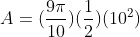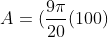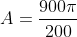Trigonometry : Area of a Sector

Example Questions

Example Question #1 : Area Of A Sector

Which of the following is the definition for a sector of a circle?

A section of a circle which is enclosed by a chord and the chord’s arc

Any section of a circle

A section of a circle which is enclosed by two radii joined at the center of the circle and the arc between the two radii

Any section of a circle that is triangular in shape

A section of a circle which is enclosed by two radii joined at the center of the circle and the arc between the two radii

Explanation:

Below is an illustration of a sector of a circle.  A sector is the area of a circle which has been enclosed by two radii and the arc between them.  A sector is not to be confused with a segment of a circle.  A segment is when the area enclosed by the chord of a circle and the arc of the chord.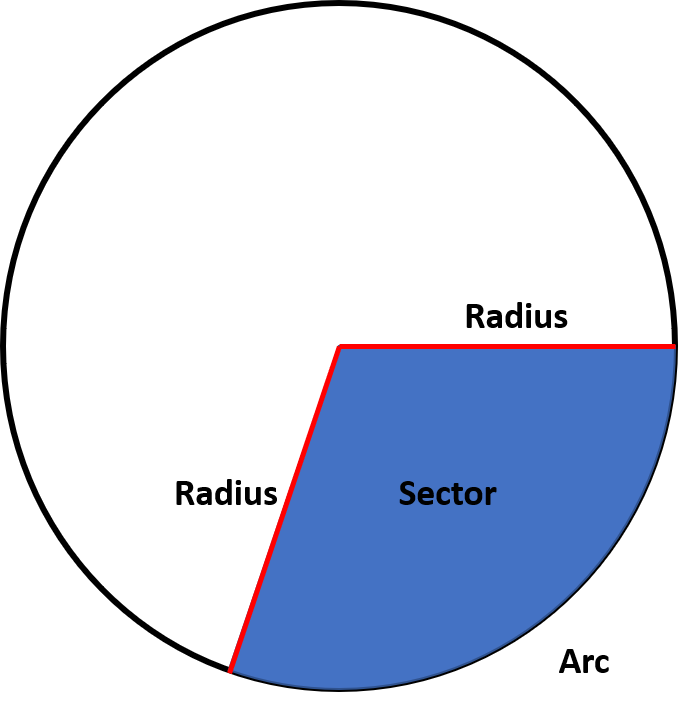Example Question #2 : Area Of A Sector

Which of the following is the formula for the area of a sector?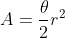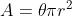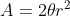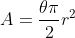Explanation:

When thinking about how to derive the formula for a sector, we must consider the angle of an entire circle.  The angle of an entire circle, 360 degrees, is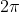and we know the area of a circle is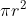.

When considering a sector, this is only a portion of the entire circle, so it is a particular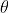out of the entire.  We can plug this into our area for a circle and it will simplify to the area of a sector.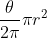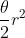Example Question #3 : Area Of A Sector

If a circle has a sector with an angle of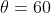and diameter of 4, what is the area of the sector?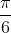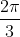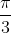Explanation:

It is always best to draw a picture in order to visualize the problem you are trying to solve.  The figure below shows the sector we are trying to find the area of.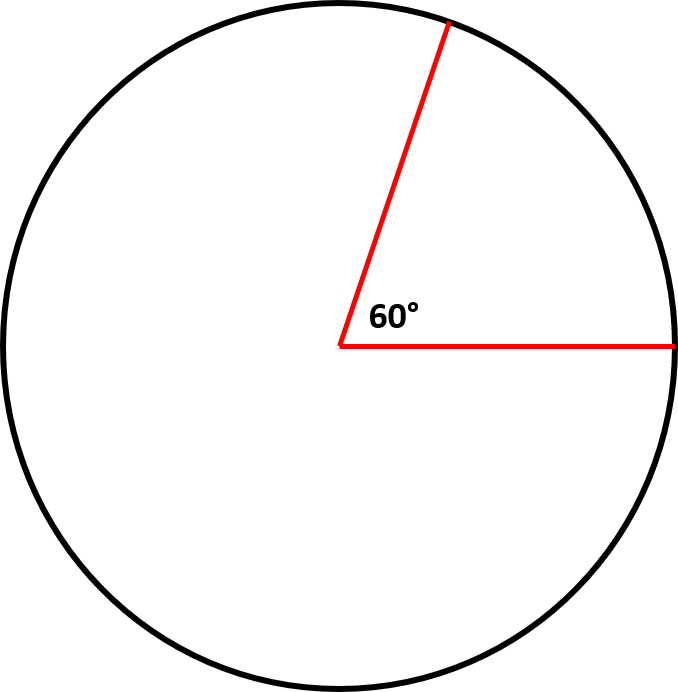We know that the formula to find the area of a sector is.  From the information given above we know that the diameter is 4.  Since we only need the radius for our formula we divide the diameter by 2 to get the radius length.  The radius has a length of 2.  We also know that we have our angle measure in degrees and must convert it to radians.  We use the conversion formula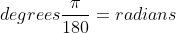.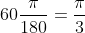Now we can plug everything into our formula and solve.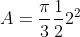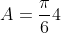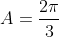Example Question #4 : Area Of A Sector

True or False: The formula to find the area of a sector only works for acute angles.

False

True

True

Explanation:

This is not true.  Even obtuse angles are less thanso this formula will still work.  We can demonstrate this using the sector below.  The radius of the circle is 6 and the obtuse angle is 330 degrees.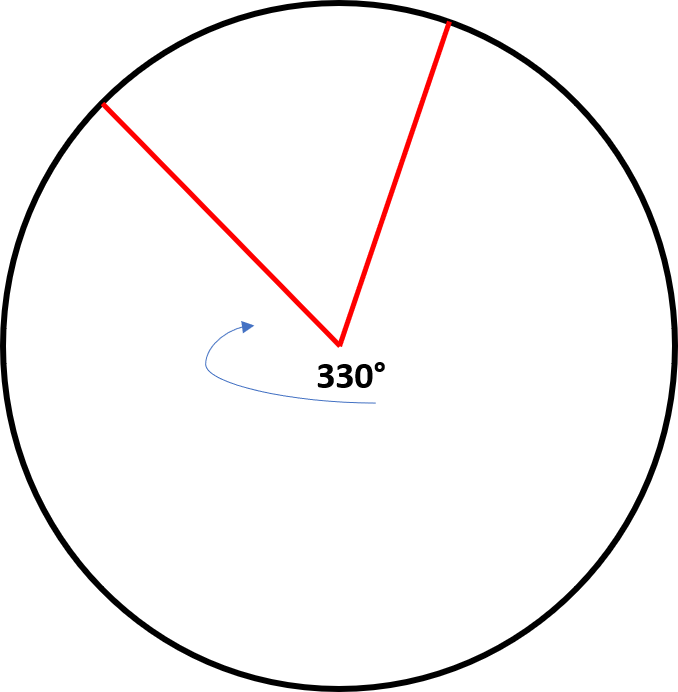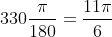We can now plug this into our formula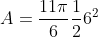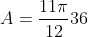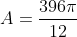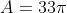Now we can confirm this to be true by computing the area of the sector formed by the

area leftover formed by the acute angle, 30 degrees.  To do this we will first find the total

area of the circle and then subtract the area of the sector formed by the acute angle.  This should be equal to the area of the larger vector if our formula works for all angles because the sum of both sectors should be the total area of the circle.

To find the area of the circle: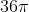To find the area of the smaller sector (note, 30 degrees in radians is: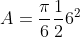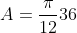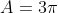Clearly, the total area of the circle minus the area of the small sector is equal to the area

of the larger circle, therefore this formula works for all angles less than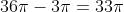Example Question #5 : Area Of A Sector

Knowing that the arc length of a sector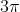and the angle measure is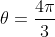, what is the area of the sector?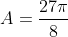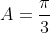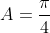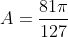Explanation:

To solve this problem we must know the formula for finding the arc length of a sector.  This formula is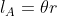.  With the given information we are able to solve for the radius which we can then use to solve for the area of the sector itself.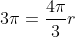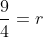Now we can plug this radius into the formula to solve for the area of a sector.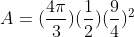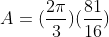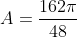Example Question #6 : Area Of A Sector

True or False: You have a sector of a circle and are given the radius of the circle and the total area of the circle.  You are able to find the area of the sector.

False

True

False

Explanation:

The formula for finding the area of a circle is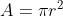.  If we know the radius and the area of the entire circle, we still do not know the angle that forms the sector or the arc length.  Therefore we do not have enough information to solve for the sector area.  In order to find the area of the sector we need to either know the angle of the sector and the radius, or have some means to solve for this information.

Example Question #7 : Area Of A Sector

A circle has a sector formed by radii of length 3 and an angle of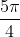.  What is the area of the sector?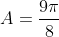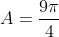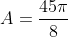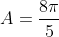Explanation:

The formula to solve for the area of a sector is.  We have all the information needed to plug the values right into the formula and solve for the area.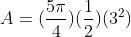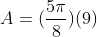Example Question #8 : Area Of A Sector

You are given a circle with a sector that is formed by an arc length of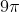.  The circle has a diameter of 20.  What is the area of the sector?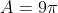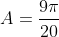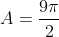Explanation:

First, if we have a diameter of 20, we know that the radius must be half of the diameter.  We have a radius length of 10.  Now, we know the arc length so we must use the arc length formula to solve for the angle measure.  To find the length of an arc of a sector we use the formula.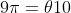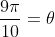By solving for the angle, we have enough information to solve for the sector area.